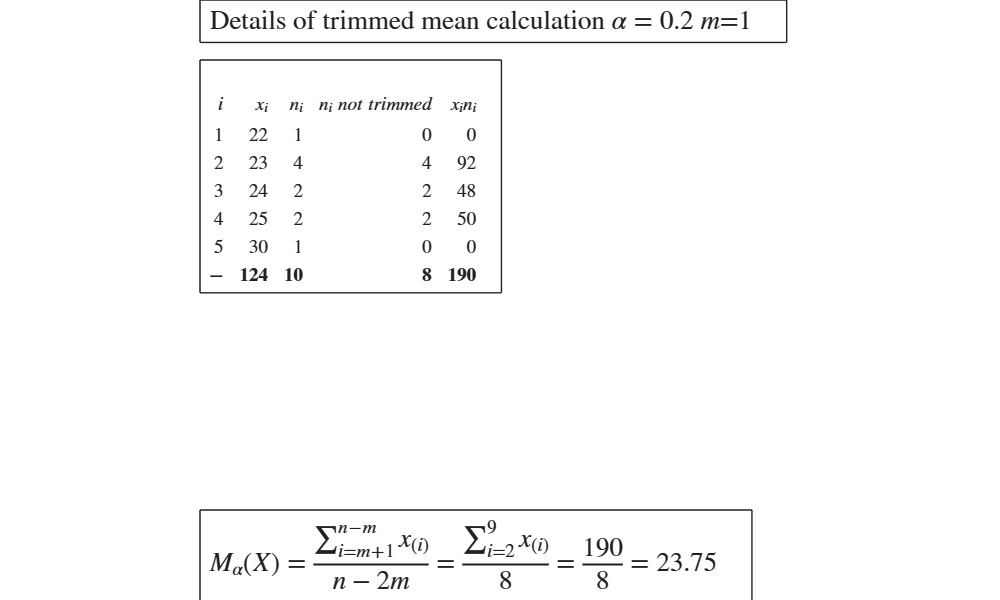# GUItrimmean

GUItrimmean shows the necessary calculations to obtain the trimmed mean in a GUI.

## Syntax

• out=GUItrimmean(x,percent, freq)example

## Description

 out =GUItrimmean(x, percent, freq) Use of trimmed mean with 'percent' as per cent of trimming.

## Examples

expand all

###Use of trimmed mean with 'percent' as per cent of trimming.

In this case we use 50% as per cent of trimming. (See page 92 of [MRZ])

x=[60 30 50 50  80 35000  80 95];
out=GUItrimmean(x,50);## Related Examples

expand all

### Example of use of trimmed mean with 25 per cent of trimming.

(See page 92 of [MRZ])

x=[60 30 50 50  80 35000  80 95];
out=GUItrimmean(x,25);

###Trimmed mean in a frequency distribution.

Matrix X below contains the frequency distribution of the grades obtained by 10 students. (See page 71 of [CMR]) Compute the truncated mean of the grades using alpha=0.2

X=[22	1
25	2
30	1
23	4
24	2];
x=X(:,1);
freq=X(:,2);
GUItrimmean(x,20,freq)
ans =

struct with fields:

data: [6×5 table]
trimmedmean: 23.7500### Comparison of truncated mean using original data and frequency distribution.

% Vector x contains the temperature (Celsius degrees) in 10 places. (See page 23 of [CMR])
% Compute the truncated mean using alpha equal to 0.4.
x=[18	24	21	19 	27	12	21	15	12	16];
trimperc=40;
GUItrimmean(x,trimperc)
% Note that the same results are obtained if you use frequency
% distribution in the firsts two columns of Ta
Ta=tabulateFS(x);
GUItrimmean(Ta(:,1),trimperc,Ta(:,2))

## Input Arguments

### x — vector of numeric data. Vector.

Vector containing strictly numerical data.

Data Types: double

### percent — trimming percent. Scalar.

Percentage of input data to be trimmed specified as a scalar between 0 and 100 (generally between 0 and 50).

For example, if x is a vector that has n values, the trimmed mean is the mean of x excluding the highest and lowest m data values, where m =[n*(percent/100)/2].

For example if n=11 and percent=30, $n*(percent/100)/2=1.65$.

$m=[1.65]=1$ therefore the smallest and largest observations are excluded from the computation.

Example - 1:10

Data Types: double

### freq — weights. Vector.

Vector with the same length of x containing the (frequencies) weights assigned to each observation.

Example - 1:10

Data Types: double

## Output Arguments

### out —detailed output to compute the index. Table

Table with n+1 rows (where n is the length of x) containing what is shown in the GUI. Last row contains the totals.

Milioli, M.A., Riani, M., Zani, S. (2019), "Introduzione all'analisi dei dati statistici (Quarta edizione ampliata)". [MRZ]

Cerioli, A., Milioli, M.A., Riani, M. (2016), "Esercizi di statistica (Quinta edizione)". [CMR]